###Suan Pan and the Unit Rod - Multiplication

##### 1.03...... one whole number - unit rod shifts 1 rod to the right. 45.003... two whole numbers - unit rod shifts 2 rods to the right....etc. 0.75....... no whole numbers, no trailing zeros, unit rod does *not* shift. 0.0125... one trailing zero - unit rod shifts 1 rod to the left. 0.003..... two trailing zeros - unit rod shifts 2 rods to the left...etc.

Using these Suan Pan techniques to determine a decimal answer is remarkably easy. In order to find the correct decimal answer one takes into account either the whole numbers or the trailing zeros in the multiplier and shifts the unit rod left or right as needed. To better illustrate this here are some decimal variations of the same problem. On a personal note I've always found using different decimal variants of the same problem to be a good way to get my head around working with decimals and unit rods.

Example 1: 14 x 25 = 350

##### ▪ Rod G x B x A. Note: the multiplicand on rod G changes to become part of the product. ▪ Rod F x B x A. Note: the multiplicand on rod F changes to become part of the product.

Step 1: Choose rod G as the unit rod. Set multiplier 25 on rods AB and multiplicand 14 on rods FG. (Fig. 1)Fig. 1 ```Step 1  A B C D E F G H I J K L M       2 5 0 0 0 1 4 0 0 0 0 0 0 Step 1 ```

Step 2: Multiply 4 by 5 and add 20 to rods HI.
Step 2a: Multiply 4 by 2 and add 08 to rods GH. Note: what was originally multiplicand 4 on G has changed to 1. (Fig. 2)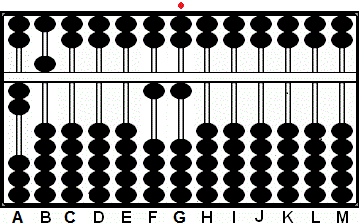Fig. 2 ```Step 2  A B C D E F G H I J K L M       2 5 0 0 0 1 4 0 0 0 0 0 0 + 2 0 Step 2   + (0)8           Step 2a 2 5 0 0 0 1 1 0 0 0 0 0 0```

Step 3: Multiply 1 by 5 and add 05 to rods GH.
Step 3a: Multiply 1 by 2 and add 02 to rods FG. (Fig. 3)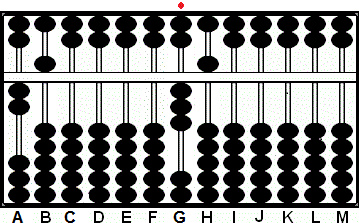Fig. 3 ```Step 3  A B C D E F G H I J K L M       2 5 0 0 0 1 1 0 0 0 0 0 0 + 0 5 Step 3 + (0)2             Step 3a 2 5 0 0 0 0 3 5 0 0 0 0 0```

Step 4 and the answer: Identify the new unit rod. Because the multiplier has two whole numbers shift the unit rod two to the right. The unit rod has now shifted to rod I leaving the answer 350 on rods GHI. (Fig. 4)Fig. 4 ```Step 4  A B C D E F G H I J K L M       Step 4 2 5 0 0 0 0 3 5 0 0 0 0 0 ```

Decimal Variations

This next problem can be done in exactly in the same way but using different decimal numbers. The only thing that changes is the position of the unit rod.

Example 2: 0.014 x 0.025 = 0.00035

Choose rod D to be the unit rod. Set multiplier 25 on rods AB and 14 on rods FG (Fig. 1)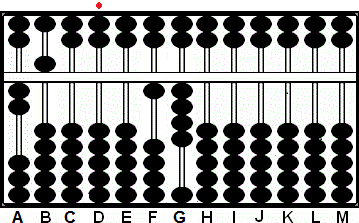Fig. 1 ```Step 1  A B C D E F G H I J K L M       2 5 0 0 0 1 4 0 0 0 0 0 0```

Continue with the problem exactly as above eventually arriving at the same answer. The only difference is the position of the unit rod is on rod D. (Fig. 2)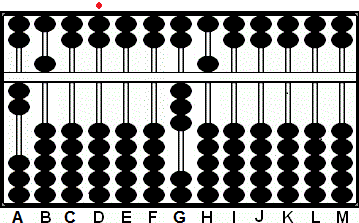Fig. 2 ```Step 2  A B C D E F G H I J K L M       2 5 0 0 0 0 3 5 0 0 0 0 0```

The final step and the answer: Identify the new unit rod. Because the multiplier 0.025 has one trailing zero shift the unit rod one to the left. The unit rod has now shifted to rod C leaving the answer 0.00035 on rods C - H. (Fig.3)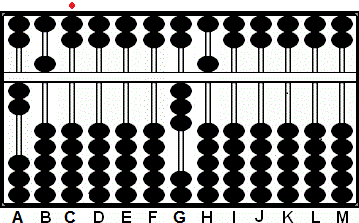Fig. 3 ```Step 3  A B C D E F G H I J K L M       2 5 0 0 0 0 3 5 0 0 0 0 0```

Other Examples

1.4 x 25 = 35
0.14 x 0.25 = 0.035
14 x 0.0025 = 0.035
0.0014 x 0.25 = 0.00035  .... etc. etc.